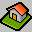PhD Dissertation

 Author Saeed Salehi Title Herbrand Consistency in Arithmetics with Bounded Induction Language English Supervisor Professor Zofia Adamowicz Reviewers (1) Professor Henryk Kotlarski (2) Professor Marcin Mostowski Date June 2002

Here is the PhD Thesis (in PDF format - 84 pages); and this is an outline of the thesis (PDF file; 4 pages).

Abstract

By Gödel's celebrated incompleteness theorems, TRUTH is not conservative over PROVABILITY in theories that contain sufficiently strong fragments of arithmetic. In other words, for any give reasonable arithmetical theory T, there exists a true arithmetical sentence GT which is not provable in T. Moreover, this formula GT can be chosen to be a Π1-formula; thus TRUTH is not even Π1-conservative over PROVABILITY in general arithmetics. Needless to say, this GT may be provable in a stronger theory (than T ). Thus, Π1-conservativity of a theory over its sufficiently strong sub-theories is an interesting, and often difficult, question in mathematical logic.

A natural candidate for showing the Π1-unconservativity of T over its sub-theory SÌT is the consistency statement of S, Con(S); i.e., one would wish to show that T |— Con(S), and then use Göodel's Second Incompleteness Theorem to infer that S |–/– Con(S). Let us recall that for Zermelo-Frankel set theory ZFC and Peano's Arithmetic PA we have ZFC |— Con(PA), though PA |–/– Con(PA). Also, if n is the fragment of PA in which the induction schema is restricted to Σn–formulas, we have n+1 |— Con(n ) and n |–/– Con(n ) for any n>0. For weak arithmetics this candidate does not work for Π1-separating 0+exp over 0: we have 0+exp |–/– Con(0 ) (and also 0 |–/– Con(0 )). In 1981, J. Paris and A. Wilkie proposed cut-free consistency statement for this purpose; though at that time it was not yet proved that 0 |–/– CFCon(0 ) where CFCon stands for cut-free consistency. However, it was known that 0+exp |— CFCon(0 ). We note that the cost of cut-elimination in proof theory is super-exponential, so in weak arithmetics cut-free provability is not equivalent to the usual (Hilbert style) provability. Indeed, in those theories CFCon is a stronger predicate than Con.

From another point of view, unprovability of cut-free consistency of weak arithmetics in themselves is an interesting generalization of Gödel's Second Incompleteness Theorem. The original proof of this theorem was presented for the usual (Hilbert) consistency predicate of theories that contain primitive recursive arithmetic. However, later on, the theorem was proved for all r.e. extensions of Robinson't Arithmetic Q. So, one direction of generalizing the theorem was investigating the boundary cases: finding the weakest possible theories whose r.e. extensions cannot prove their own consistency. Another direction could be weakening the consistency predicate in addition to weakening the underlying theory. By 1985, another 0+exp-provable Π1-sentence that is unprovable in 0 had been found; however the question of the unprovability of cut-free consistency in theories weaker than 0+exp remained open (see Pudlák's paper where he mentions the problem explicitly for Herbrand consistency in 1985). Let us recall that Herbrand consistency of a theory is the propositional satisfiability of every (finite) set of its Skolem instances. Herbrand consistency of a theory T is denoted by HCon(T).

The first demonstration of the unprovability of cut-free consistency in weak arithmetics was made by Z. Adamowicz who proved in an unpublished manuscript in 1999 (later appeared as a technical report) that the Tableau-consistency of 01 is not provable in itself. Later on with P. Zbierski (2001) she proved the theorem (Gödel's Second Incompleteness Theorem) for Herbrand consistency of 02, and a bit later she gave a model theoretic proof of it (2002). Extending these results for 0 was proposed to me as a topic for my PhD thesis by her.

By modifying the definition of Herbrand consistency, Z. Adamowicz's model-theoretic proof is generalized to 01 in Chapter 5 of the thesis (the result is not published anywhere else). Also, it is shown in Chapter 3 that 0 |–/– HCon(0) where the theory 0 is axiomatized by a conventional axiomatization of 0 augmented with two 0-provable sentences. These results were presented in the Logic Colloquium 2001 Vienna, Austria, and in the student session of the European Summer School in Logic, Language, and Information, 2001 Helsinki, Finland; a paper is published in the proceedings of the summer school. Chapter 4 proves 0 |–/– HCon(0 ) where Ω expresses the totality of ω(x)=xlog log x; here the conventional axiomatization of 0 is taken in the proof. The theory 0 lies between 0 and 01 .
In the end of Z. Adamowicz & P. Zbierski's paper (2001) three questions were asked. In Chapter 5 the second question is answered negatively, by elaborating a concrete counter-example (introduced in Chapter 2).

Independently, D. Willard (2002) introduced an 0-provable Π1-formula V and showed that any theory whose axioms contains Q+V cannot prove its own Tableaux consistency. He also showed that Tableaux consistency of 0 is not provable in itself; this proved the conjecture of J. Paris & A. Wilkie mentioned above.Back to Home-Page